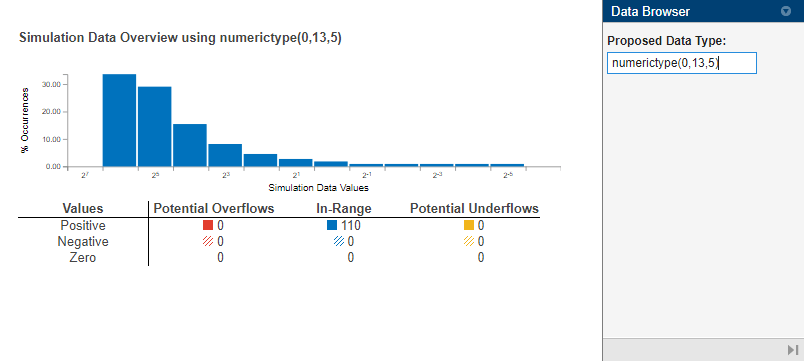# show

Open `NumericTypeScope` object

## Syntax

``show(H)``

## Description

example

````show(H)` opens the `NumericTypeScope` object `H` and brings it into view. Closing the scope window does not delete the object from your workspace. If the scope object still exists in your workspace, you can open it and bring it back into view using the `show` function.```

## Examples

collapse all

Use the `NumericTypeScope` to view the dynamic range of a `fi` object.

Create a `fi` object and set the `DataTypeOverride` to `ScaledDoubles`.

```a = fi(magic(10),1,8,2); b = fi([a; 2.^(-5:4)],1,8,3); fp = fipref; initialDTOSetting = fp.DataTypeOverride; fp.DataTypeOverride = 'ScaledDoubles'; ```

Create a `NumericTypeScope` object. You can use the `reset` method to ensure that all stored information is cleared from the `NumericTypeScope` object `h`.

```h = NumericTypeScope; reset(h) ```

Use the `step` method to process your data and visualize the dynamic range of the `fi` object `b`.

```step(h,b); ```Closing the `NumericTypeScope` window does not delete the object from your workspace. Close the `NumericTypeScope` window and reopen it using the `show` function.

```show(h); ```

The `NumericTypeScope` displays a `log2` histogram which shows that the values appear both outside of the range and below the precision of the data type of the variable. Pause on one bar of the histogram to view the percentage of the total values that are represented by that bar.In this case, the data type of `b` is `numerictype(1,8,3)`. The `numerictype(1,8,3)` data type provides 5 integer bits, including the signed bit, and 3 fractional bits. Thus, this data type can represent only values between -2^4 and 2^4 - 2^-3 (from -16 to 15.8750). Given the range and precision of this data type, values greater than 2^4 fall outside the range and values less than 2^-3 fall below the precision of the data type.

The `NumericTypeScope` shows that values requiring bits 5, 6, and 7 are outside the range and values requiring fractional bits 4 and 5 are below precision. Given this information, you can prevent values that are outside range and below precision by changing the data type of the variable `b` to `numerictype(0,13,5)`.

Given this information, you can prevent values that are outside range and below precision by changing the data type of the variable `b` to `numerictype(0,13,5)`. In the `NumericTypeScope`, enter `numerictype(0,13,5)` in the Proposed Data Type box.```fp.DataTypeOverride = initialDTOSetting; ```

## Input Arguments

collapse all

`NumericTypeScope` object, specified as a `NumericTypeScope` object.

Example: `show(H)`

## Version History

Introduced in R2010a